# Single vs. multiple propeller efficiency

Posted by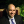DusanIntroduction

A lot of new personal flying devices are gaining interest in the media lately, Almost all are a variant of multicopters configuration. The idea behind multicopters is the simplicity of control, by varying the power to the corresponding motor; and the redundancy, if there are 8 motors, and one fails, it is easy to have the other 7 to take the slack. One example of such multicopter is the Ehang 184 passenger droneEhang 184 passenger drone

Although there is the impression that multicopters are aerodynamically less efficient than single rotor configuration - e.g. the classical helicopter, I had trouble finding a document stating that for the same aerodynamic conditions: disk loading, tip airspeed and solidity; more propellers are less efficient than a single propeller.

During my research into this subject I stumbled upon a quad-copter having a unusual configuration, one big propeller to efficiently create lift and 3 smaller rotors to provide control. This design kind of confirms my supposition, the authors claim it improves hover expended energy by 20%: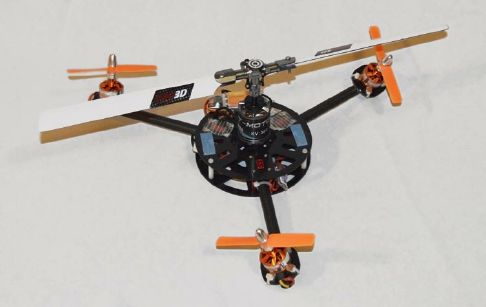Analysis

To get more insight about the efficiency of one large rotor compared to multiple smaller rotors, I’m doing an analysis of a single rotor (disregarding the anti-torque mechanism) and comparing it with a quad, having 4 similar rotors. The devices are considered to have the same weight, disk loading, tip air speed and solidity.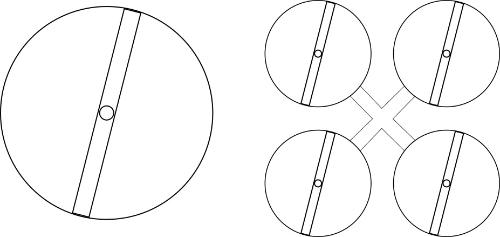Having the same weight and the same disk loading, the disk area of the single rotor is equal to the total disk area of the quad.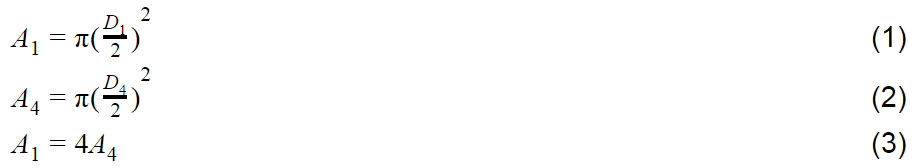From (1), (2) and (3)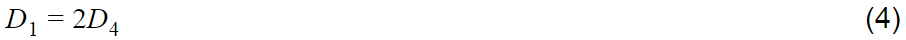Having the same disk area, simple applied geometry gives that the diameter of the single rotor, is two times larger than the diameter of rotors of the quad, assuming the quad rotors are equal.

Same solidity and same disk area means the total blade area is the same, soFrom (4 ) and (5)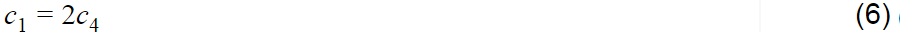The chord of the single rotor blade is double the chord of one rotor of the quad.

As the tip air speed is the same, then the air speeds along the blade are similar. The single aerodynamic difference in the flow is given by the Reynolds number, which is half for the quad, as its blade chord is half of the single rotor chord.

The friction depends a lot on the Reynolds number. If you are not familiar with Reynolds number, it expresses the inertia and viscosity ratio for a particular flow. More details in here:

https://en.wikipedia.org/wiki/Reynolds_number

The flow along the blade chord transitions at some point from laminar to turbulent

Looking at the friction coefficient, it depends a lot on the Reynolds number; for laminar flow, the Blasius solution expresses the coefficient of friction variation with Reynolds number: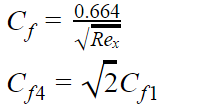The difference in Reynolds numbers for the single rotor blade and the quad blade means that laminar friction coefficient is 41% larger for the quad rotors.

For turbulent flow, the friction coefficient is somewhat less influenced, the Prandtl's one-seventh-power law expression:Doing the math, gives that the friction coefficient for turbulent flow is 10.4% larger for the quad rotors.

It can be concluded that the friction is somewhere between 10.4 to 41% larger for the quad compared to the single rotor.

Airfoil data

Now let's look at the generated lift; for this we'll compare the airfoils characteristics for Reynolds numbers of 500,000 and 1,000,000. A typical rotor airfoil is the NACA0012.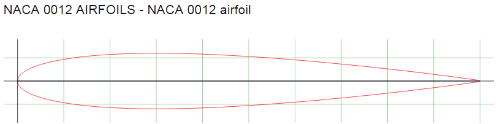Extracting data from http://airfoiltools.com/airfoil/details?airfoil=n0012-il#polars
The L/D at Re of 500,000 and 1,000,000 are 61.7 and respectively 75.6; a decrease of L/D of 22.5%. This means for the same lift, the drag is increased by 22.5%, in good concordance with the previous friction coefficient calculations.

The same analysis can be done for more than 4 rotors, as expected the results are worse. As for example, the Reynolds number reduces 2.82 times for 8 rotors. For the NACA 0012 the L/D degrades by 59%, for the same lift the drag is almost 60% larger!

Conclusion

In conclusion,  the more rotors an aircraft have, the less efficient it’s hovering is. Not sure how the Lilium Jet having 36 ducted rotors can have any hovering efficiency, indeed from published data, it needs 320KW (430hp) to hover.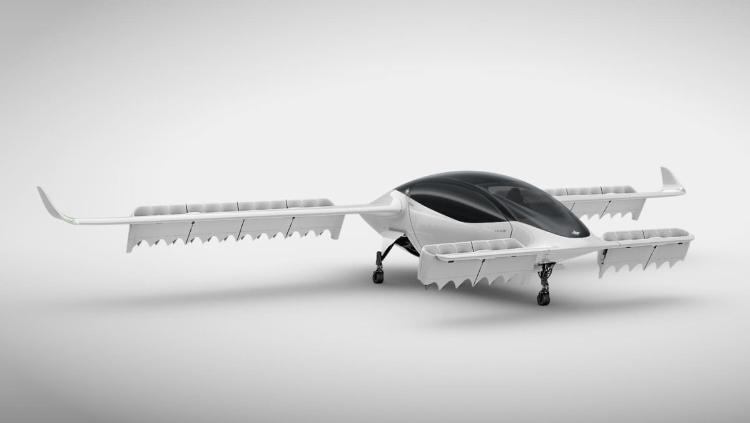As an example, compare these personal VTOL vehicles:

Ehang 184
Configuration: 8 rotor multicopter
Rotors: 8 x 1.5m diameter (Area=14.13m2)
Motor/Engine: 8 x 13.25KW = 106KW (142hp)
Weight: 360Kg

Hiller VZ1 Pawnee
Configuration: Counter rotating ducted prop
Rotors: 2 x 2.13m diameter (Area=7.12m2)
Motor/Engine: 2 x 30KW = 60KW (80hp)
Weight: 251.7Kg

Mosquito Aviation XE
Configuration: Classic helicopter
Rotors: 1 rotor 2 bladed 5.95m (Area=27.8m2)
Motor/Engine: 40KW (64hp)
Weight: 280Kg

The lowest power required is for the single rotor helicopter, due to its low disk loading. The Ehang 184 needs 76% more power than the Hiller VZ1 Pawnee, even that the Ehang 184 having a lower disk loading.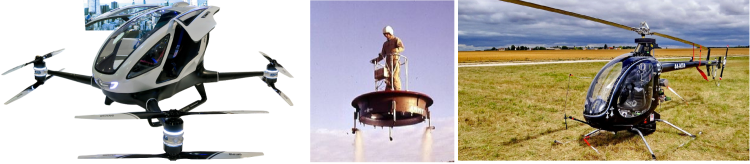## 1 CommentClassicListThreaded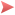Propeller charts prove this to be the case. Large Propeller (from McCormick)Small Propeller (from Brandt and Selig, "Propeller Performance Data at Low Reynolds Numbers")Note the difference in thrust coefficient and the advance ratio range.